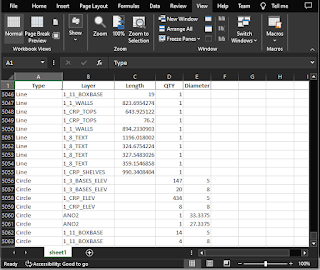## Wednesday, November 29, 2023

### Pandas for data extraction, merging DataFrames, Python for AutoCAD

I explored Groupby and Aggregate here

import traceback
import PyRx as Rx
import PyGe as Ge
import PyGi as Gi
import PyDb as Db
import PyAp as Ap
import PyEd as Ed

import pandas as pd

def PyRxCmd_doit():
try:
#setup dictionaries to hold our data
lineMap = {"Type": [], "Layer": [], "Length": [], "QTY": []}
circleMap = {"Type": [], "Layer": [], "Diameter": [], "QTY": []}

#current database
db = Db.curDb()
model = Db.BlockTableRecord(db.modelSpaceId())

#search model for all lines (derived from)
lineIds = model.objectIds(Db.Line.desc())
for id in lineIds:
line = Db.Line(id)
lineMap["Type"].append("Line")
lineMap["Layer"].append(line.layer())
lineMap["Length"].append(line.startPoint().distanceTo(line.endPoint()))
lineMap["QTY"].append(1)

#search model for all circles (derived from)
circleIds = model.objectIds(Db.Circle.desc())
for id in circleIds:
circle = Db.Circle(id)
circleMap["Type"].append("Circle")
circleMap["Layer"].append(circle.layer())
circleMap["Diameter"].append(circle.diameter())
circleMap["QTY"].append(1)

#create the DataFrames
lfd = pd.DataFrame(lineMap)
cfd = pd.DataFrame(circleMap)

# called before concat because the column names are different
# groupby & aggregate
lfd = (lfd.groupby(["Type", "Layer", "Length"],
sort=False, as_index=False).agg({"QTY": "sum"}))

# groupby & aggregate
cfd = (cfd.groupby(["Type", "Layer", "Diameter"],
sort=False, as_index=False).agg({"QTY": "sum"}))

# concat the DataFrames
result = pd.concat([lfd, cfd])

# write to excel, but don't write the index
with pd.ExcelWriter("e:\\pandas_to_excel.xlsx") as writer:
result.to_excel(writer, sheet_name="sheet1", index=False)

except Exception as err:
traceback.print_exception(err)

## Friday, November 24, 2023

### Generate plot chart from an AutoCAD table using Python for AutoCAD

The tools we need are matplotlib and pandas

Step 1, is to create a pandas dataframe from an AutoCAD table, the structure is a dictionary {header : [data array]}. We’ll need to create a map (dictionary) of our headers so we can access them later. Next, iterate the data cells and put them in the appropriate bucket

Step 2, setup our plot configuration, setup our colors. Note, we need to make sure the column we want to plot is the correct data type, if you’ll notice the astype cast. Run the plot

Optional step 3, insert the result as a raster image

here's the code

import PyRx as Rx
import PyGe as Ge
import PyGi as Gi
import PyDb as Db
import PyAp as Ap
import PyEd as Ed
import traceback

import matplotlib.pyplot as plt
from matplotlib.pyplot import savefig
import numpy as np
import pandas as pd

# create a dict to hold the headers
# create a dataframe {header : [data]}
def createDataFromTable(id : Db.ObjectId):

data = {}
table = Db.Table(id)

# save header row so we can lookup the header name while looping the rows
range = table.cellRange()
range.topRow = 1
range.bottomRow = 1

for cell in table.getIterator(range):
key = table.textStringFmt(cell, Db.FormatOption.kIgnoreMtextFormat)
data[key] = []

# iterate data rows, an put the data in our data map
range = table.cellRange()
range.topRow = 2

for cell in table.getIterator(range):
value = table.textStringFmt(cell, Db.FormatOption.kIgnoreMtextFormat)
data[key].append(value)

return pd.DataFrame(data)

def PyRxCmd_doit():
try:
es = Ed.Editor.entSel("\nSelect a table: ", Db.Table.desc())
if es != Ed.PromptStatus.eNormal:
print("oof")
return

params = {
"text.color": "crimson",
"xtick.color": "crimson",
"ytick.color": "crimson",
"axes.edgecolor": "crimson",
"axes.labelcolor": "crimson",
"figure.facecolor": "crimson"}

plt.rcParams.update(params)

plt.xlabel("Item")
plt.ylabel("Cost")
plt.title("Cost per item")

df = createDataFromTable(es)
df['Unit']=df['Unit'].astype(float)

df["Unit"].plot.bar()
savefig("demo.png", transparent=True)
plt.clf()# clear

#todo insert as image
print("yay")
except Exception as err:
traceback.print_exception(err)

## Thursday, November 23, 2023

### Using matplotlib in Python for AutoCAD

There’s a couple of options when showing a plot from matplotlib,

1, is to use wxPython. This is nice because you can embed the plot with other controls, you also have the option of making the window modal, or put the plot in a palette.  There’s samples here and here

2, Is you just want to show the plot. Maybe you want to save the plot as a PNG and paste in into AutoCAD as a Raster image.

In this case, all you really need to do is make sure you call show with block=False

import PyRx as Rx
import PyGe as Ge
import PyGi as Gi
import PyDb as Db
import PyAp as Ap
import PyEd as Ed
import traceback

import matplotlib.pyplot as plt
import numpy as np

def PyRxCmd_doit():
try:
x = np.linspace(0.1, 2 * np.pi, 41)
y = np.exp(np.sin(x))

plt.stem(x, y)
plt.show(block=False) #don't block

except Exception as err:
traceback.print_exception(err)

### Pandas for data extraction, merging DataFrames, Python for AutoCAD

I explored Groupby and Aggregate here import traceback import PyRx as Rx import PyGe as Ge import PyGi as Gi import PyDb as Db ...